/

### Function Description

Returns the (sample) kurtosis,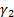(or more precisely the ‘excess’ kurtosis) of a series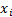(for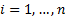), calculated as follows: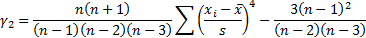Here,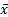is the mean andis the (sample) standard deviation of the series

The equivalent function in Microsoft Excel is KURT.

Contents | Prev | Next